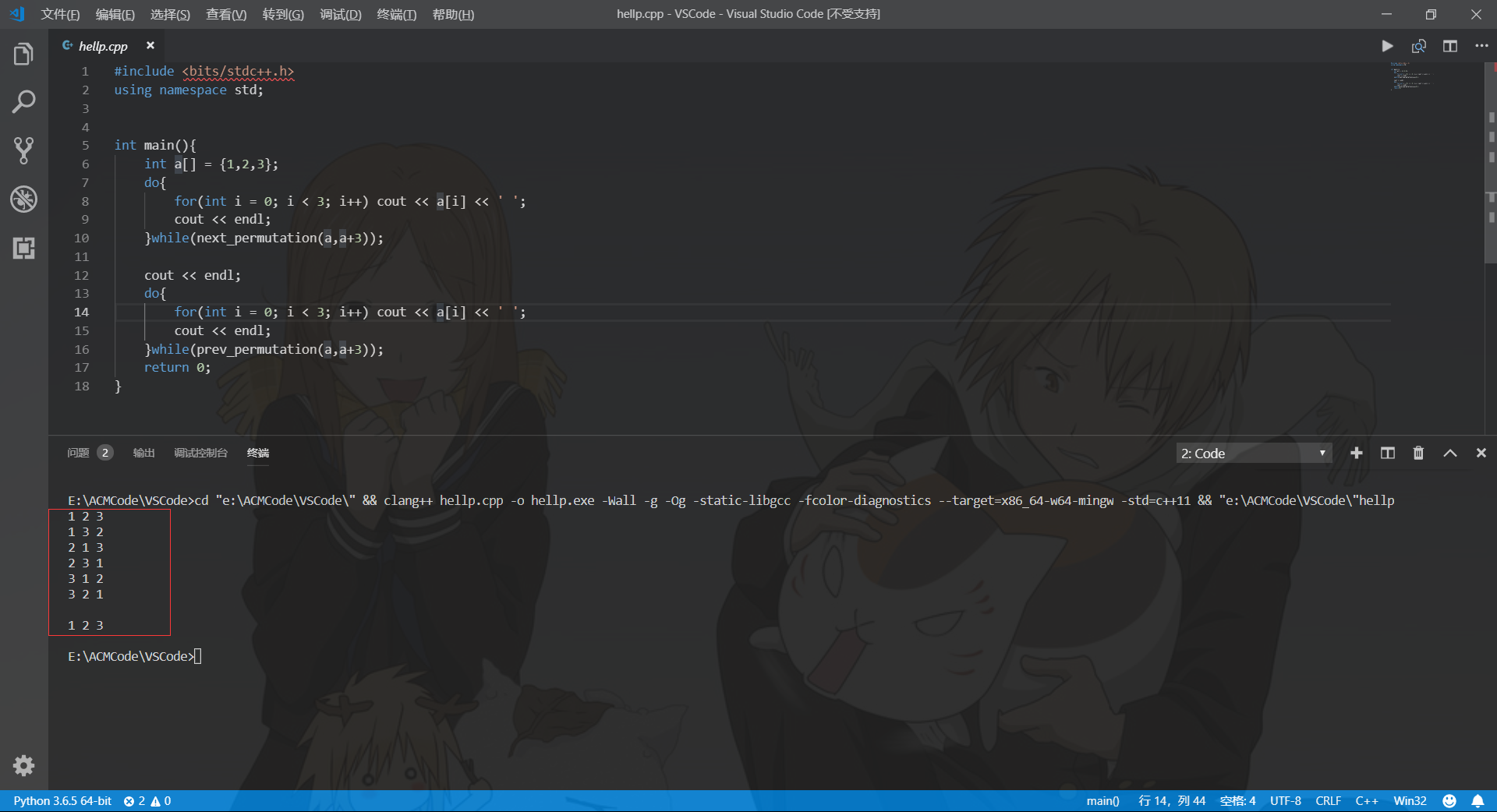# STL中关于全排列next_permutation以及prev_permutation的用法

这两个函数都包含在algorithm库中。STL提供了两个用来计算排列组合关系的算法，分别是next_permutation和prev_permutation。

# 一、函数原型

• next_permutation:
1 template< class BidirIt >bool next_permutation( BidirIt first, BidirIt last );
2 template< class BidirIt, class Compare >bool next_permutation( BidirIt first, BidirIt last, Compare comp );

• prev_permutation:
1 template< class BidirIt >bool prev_permutation( BidirIt first, BidirIt last);
2 template< class BidirIt, class Compare >bool prev_permutation( BidirIt first, BidirIt last, Compare comp);

## 1.参数

first，end ——重新排序的元素范围

comp —— 自定义比较函数

• abc
• acb
• bac
• bca
• cab
• cba

# 二、代码演示

 1 #include <bits/stdc++.h>
2 using namespace std;
3
4
5 int main(){
6     int a[] = {1,2,3};
7     do{
8         for(int i = 0; i < 3; i++) cout << a[i] << ' ';
9         cout << endl;
10     }while(next_permutation(a,a+3));
11
12     cout << endl;
13     do{
14         for(int i = 0; i < 3; i++) cout << a[i] << ' ';
15         cout << endl;
16     }while(prev_permutation(a,a+3));
17     return 0;
18 }

预计上面代码的运行结果应该是输出两组1，2，3的排列组合方式，共12行，但实际运行结果如下：Why？

# 三、手动实现

 1 template<class T>
2 bool next_permutation(T begin, T end){
3     T i = end;
4     if (begin == end || begin == --i) return false;
5
6     while(1){
7         T i1 = i,i2;
8         if (*--i < *i1) {   //找第k位
9             for(i2 = end; *i >= *i2; i2--);//从后往前找到逆序中大于*i的元素的最小元素
10             iter_swap(i, i2);
11             reverse(i1, end);////将尾部的逆序变成正序
12             return true;
13         }
14         if (i == begin) {
15             reverse(begin, end);
16             return false;
17         }
18     }
19 }

prev_permutation的代码实现也类似，这里不再给出，各位可以自行尝试。

# 四、复杂度分析

posted @ 2018-12-28 23:51  Xenny  阅读(703)  评论(0编辑  收藏